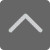TOP

# 深圳九年级上册数学一元二次方程的根与系数的关系知识点

2019-03-11 11:45:50 　来源：网络

公元前300年左右，古希腊的欧几里得(Euclid)(约前330年～前275年)提出了用一种更抽象的几何方法求解二次方程。古希腊的丢番图(Diophantus)(246～330)在解一元二次方程的过程中，却只取二次方程的一个正根，即使遇到两个都是正根的情况，他亦只取其中之一，以下小编给大家整理了深圳九年级上册数学一元二次方程的根与系数的关系知识点，希望对大家有帮助!深圳九年级上册数学一元二次方程的根与系数的关系知识点

1.x1,x2是方程x方+3x+1=0的两实数根，

即x1^2+3x1+1=0

x1^2+3x1=-1

x1^2=-3x1-1

根据根与系数关系得x1+x2=-3

x1^3+8x2+20

=x1*x1^2+8x2+20

=x1*(-3x1-1)+8x2+20

=-3x1^2-x1+8x2+20

=-3x1^2-9x1+8x1+8x2+20

=-3(x1^2+3x1)+8(x1+x2)+20

=-3*(-1)+8*(-3)+20

=3-24+20

=-1

2.根据根与系数关系，

{x1+x2=m+2是整数

{x1 x2=4m>0

所以m是正整数。

根据二次方程根的判别式，

判别式=(m+2)^2-4*4m

=m^2-12m+4

是整数，设其等于n^2(n是正整数，[负数与正数效果是一样的])

则有：(m-6)^2-32=n^2

所以(m-6)^2-n^2=32

所以(m-6+n)(m-6-n)=32

又因为m-6+n、m-6-n奇偶性相同，

且m-6+n>m-6-n

所以

{m-6+n=16,8

{m-6-n= 2,4

所以

{m+n=22或14

{m-n= 8或10

所以

m=15或12

n= 2或4

所以x^2-17x+60=0或x^2-14x+48=0

所以(x-5)(x-12)=0或(x-6)(x-8)=0

所以x1=5,x2=12,斜边长=13，m=15

或

x1=6,x2=8,斜边长=10，m=12

答：三边长为5，12，13，m=15

或三边长为6，8，10，m=12.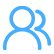请加入QQ群，试题资料免费放送！
 深圳小学交流群：254317299 一键加群 深圳初中交流群：90482695 一键加群 高一年级交流群：293049985 一键加群 高中交流群：175743089 一键加群 高二年级交流群：148082199 一键加群 2021高考交流群：644059693 一键加群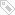标签：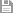保存　｜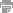打印　｜关闭